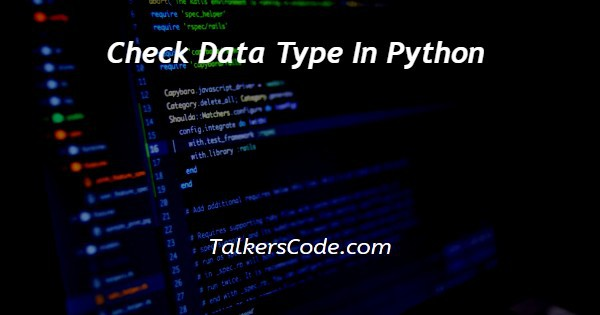# Check Data Type In Python

Last Updated : May 24, 2023In this article we will show you the solution of check data type in python, built-in Python functions make it easy to automate a wide range of tasks. The purpose of this tutorial is to show how to check the data type of a variable using python's type() function.

Python's type() function comes in handy when we want to check the data-type of a variable during programming.

Python type() helps you find the class type of a variable given as input by utilizing its built-in functionality.

Python returns the type of a variable when you place the name of the variable inside the type() function.

For debugging, it is commonly used. A type() function can be passed three arguments, such as type(name, bases, dict). We will now discuss the idea of check data type in python with example.

## Step By Step Guide On Check Data Type In Python :-

```#taking input
str = 'Python pool'
print('Datatype : ',type(str))
num = 100
print('Datatype : ',type(num))
neg = -20
print('Datatype : ',type(neg))
float = 3.14
print('Datatype : ',type(float))
complex = 2 + 3j
print('Datatype : ',type(complex))
lst = [1,2,3,4,5]
print('Datatype : ',type(lst))
Tuple = (1,2,3,4,5)
print('Datatype : ',type(Tuple))
Dict = {"a" : 2, "b" :3, "c" :1}
print('Datatype : ',type(Dict))
set = {'a', 'b', 'c', 'd'}
print('Datatype : ',type(set))```
1. The string variable "str" is declared and initialized with "Python pool".
2. Variable "str" data type can be displayed using the "type" function.
3. There is a variable called "num" declared in this file and it is initialized to 100 in the corresponding code.
4. In this code, the variable "num" is printed as its data type using the function "type".
5. The variable "neg" is declared with the value of -20, and is initialized with it.
6. A data type for the variable "neg" can be found by using the "type" function.
7. As a first step, we declare a float variable "float" and initialize it with the value 3.14.
8. A "type" function is used to verify the variable "float" is of the proper data type.
9. Using the value of 2+3j as the initial value, we declare a complex variable "complex" and initialize it with the same number.
10. In this case, we use the "type" function in order to display the data type of the variable "complex.".
11. Having declared a list variable "lst" and initializing it with a list of integers, we can now work with it.
12. By using the "type" function, we determine the data type of the variable "lst".
13. Our output consists of a tuple of integers declared in the variable "Tuple".
14. This example prints out the type of the variable "Tuple" by using the "type" function.
15. In this example, we are declaring a dictionary variable called Dict and initializing it.
16. In this example, we display the data type of the variable "Dict" using the "type" function.
17. An initial set of values is assigned to the set variable "set".
18. By using the "type" function, we display the data type of the variable "set.".

## Conclusion :-

As a result, we have successfully learned how to check data type in python.

Our objective in this tutorial was to learn how to use type() with two different parameters to check the data type of a variable.

I hope this article on check data type in python helps you and the steps and method mentioned above are easy to follow and implement.